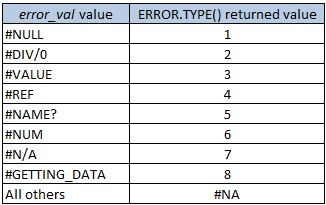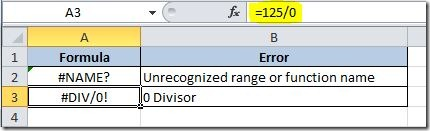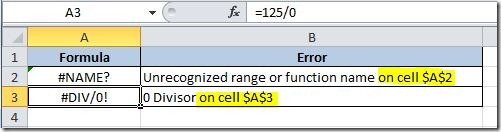### ERROR.TYPE() Function

This function returns a value corresponding to the type of Excel error. This is the syntax:

ERROR.TYPE(error_val)

error_val is a reference to a cell that has a formula that you want to check if it returns an error value. Here are the possible return values:This function can be very useful to check if a certain formula returns an error, so that you can display a custom error message to the user. For that you can use the IF() function combined with the CHOOSE() function, like this:

=IF(ERROR.TYPE(A2)<=8,CHOOSE(ERROR.TYPE(A2),"Ranges not intersecting","0 Divisor","Wrong data type","Invalid cell reference", "Unrecognized range or function name","Number error","Inappropriate function argument","Waiting for query data"),"")

This formula will check if there is an error on cell A2 formula and if it returns a ERROR.TYPE value will choose the appropriate error message to display on the cell where this formula is placed. If there is no error, it will return nothing. Here are some examples of the use of it:You can improve this formula to give you the cell address where you have the error by using the CELL() function, like this:

=IF(ERROR.TYPE(A3)<=8,CHOOSE(ERROR.TYPE(A3),"Ranges not intersecting","0 Divisor","Wrong data type","Invalid cell reference", "Unrecognized range or function name","Number error","Inappropriate function argument","Waiting for query data")& " on cell " & CELL("address",A3),"")

This will return a message like this instead:Hope this is useful to get your worksheets more user friendly.